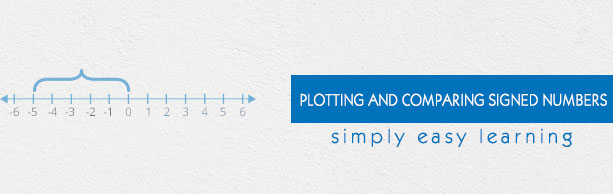# Plotting and Comparing Signed Numbers

This tutorial provides comprehensive coverage of plotting and comparing signed numbers based on Common Core (CCSS) and State Standards and its prerequisites. Students can navigate learning paths based on their level of readiness. Institutional users may customize the scope and sequence to meet curricular needs. This simple tutorial uses appropriate examples to help you understand plotting and comparing signed numbers in a general and quick way.

# Audience

This tutorial has been prepared for beginners to help them understand the basics of plotting and comparing signed numbers. After completing this tutorial, you will find yourself at a moderate level of expertise in plotting and comparing signed numbers, from where you can advance further.

# Prerequisites

Before proceeding with this tutorial, you need a basic knowledge of elementary math concepts such as number sense, addition, subtraction, multiplication, division, whole numbers, fractions, decimals, comparing and ordering whole numbers, opposite numbers, number line and so on.